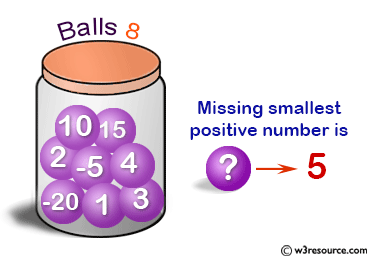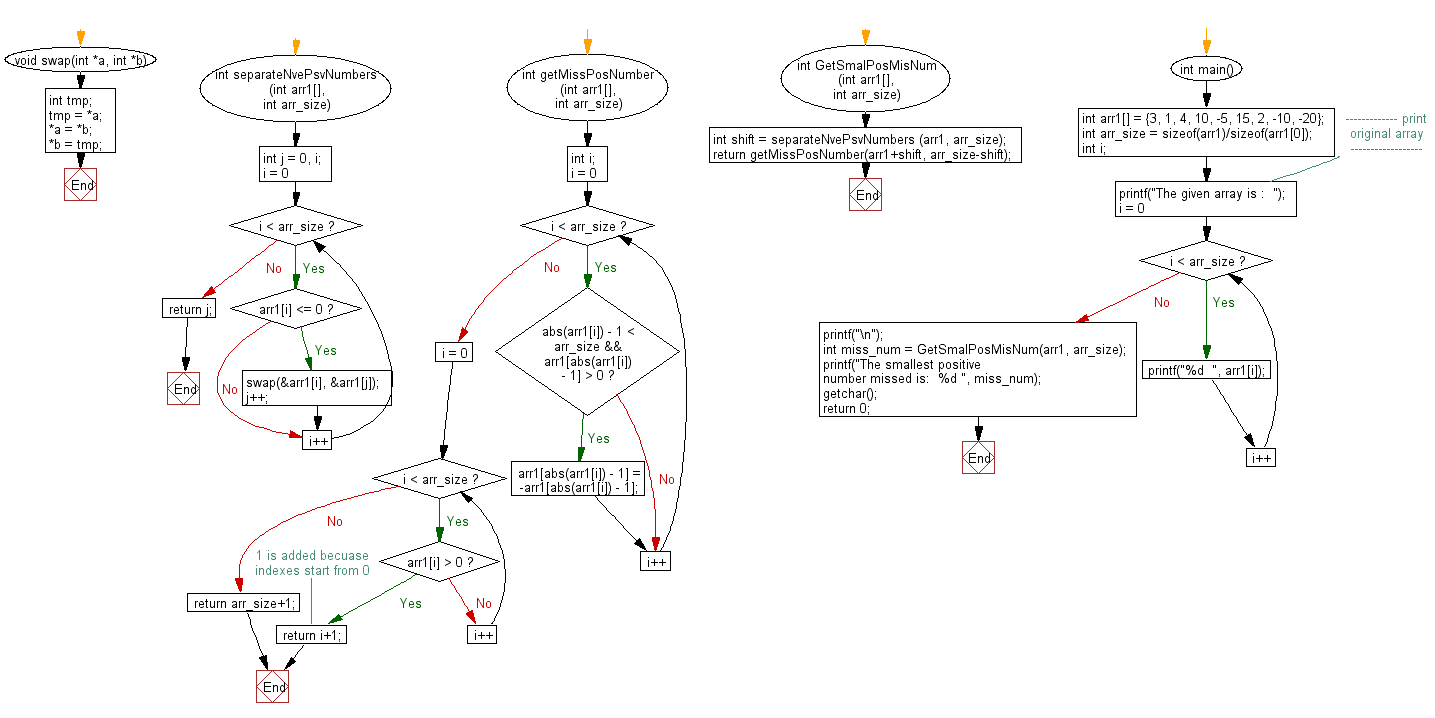﻿ C exercises: Find the smallest positive number missing from an unsorted array - w3resource# C Exercises: Find the smallest positive number missing from an unsorted array

## C Array: Exercise-46 with Solution

Write a program in C to find the smallest positive number missing from an unsorted array.

Pictorial Presentation:Sample Solution:

C Code:

``````#include <stdio.h>
#include <stdlib.h>

void swap(int *a, int *b)
{
int tmp;
tmp = *a;
*a   = *b;
*b   = tmp;
}

int separateNvePsvNumbers (int arr1[], int arr_size)
{
int j = 0, i;
for(i = 0; i < arr_size; i++)
{
if (arr1[i] <= 0)
{
swap(&arr1[i], &arr1[j]);
j++;
}
}
return j;
}
int getMissPosNumber (int arr1[], int arr_size)
{
int i;
for(i = 0; i < arr_size; i++)
{
if(abs(arr1[i]) - 1 < arr_size && arr1[abs(arr1[i]) - 1] > 0)
arr1[abs(arr1[i]) - 1] = -arr1[abs(arr1[i]) - 1];
}
for(i = 0; i < arr_size; i++)
if (arr1[i] > 0)
return i+1;  // 1 is added becuase indexes start from 0
return arr_size+1;
}
int GetSmalPosMisNum (int arr1[], int arr_size)
{
int shift = separateNvePsvNumbers (arr1, arr_size);
return getMissPosNumber(arr1+shift, arr_size-shift);
}
int main()
{
int arr1[] = {3, 1, 4, 10, -5, 15, 2, -10, -20};
int arr_size = sizeof(arr1)/sizeof(arr1);
int i;
//------------- print original array ------------------
printf("The given array is :  ");
for(i = 0; i < arr_size; i++)
{
printf("%d  ", arr1[i]);
}
printf("\n");
//------------------------------------------------------
int miss_num = GetSmalPosMisNum(arr1, arr_size);
printf("The smallest positive number missed is:  %d ", miss_num);
getchar();
return 0;
}
```
```

Sample Output:

```The given array is :  3  1  4  10  -5  15  2  -10  -20
The smallest positive number missed is:  5
```

Flowchart:C Programming Code Editor:

Improve this sample solution and post your code through Disqus.

What is the difficulty level of this exercise?

﻿